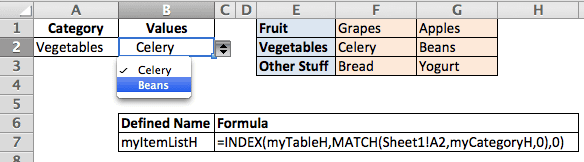# A Dynamic Dependent Drop Down List with a Horizontal Table Reference

I received a comment asking if a dynamic dependent drop-down list in Excel could have a list where the “table headers were actually rows and not columns?” Since I’ve already detailed how this is done in the article mentioned above, I’ll keep this short. The screen shot below is what I’ll be referencing. At the end of the post I’ll give a link to the file I used.

## Conditional Drop Down List (Excel)There are two named ranges,

1. 1)

myCategoryH

1. that refers to the range E1:E3 and

1. 2)

myTableH

that refers to range F1:G3.

A defined name, myItemListH, is created with the following formula =INDEX(myTableH,MATCH(Sheet1!A2,myCategoryH,0),0) that will return a row that is matched by the contents of cell A2.

In cell A2, add a Data Validation list with the source being =myCategoryH. In cell B2, add a Data Validation list with the source being =myItemListH in the conditional drop down list from Excel.

Now you’re done.

Cell A2 will give you a drop-down list of Fruit, Vegetables, or Other Stuff. And cell B2 will read the value in cell A2, match and return the proper row number, and return an array of values for that row.

### Two Ways to Use the INDEX Function to Return an Array

This is a simple case of using the INDEX function in a slightly different way. Normally, to return column 2 of a named range, you would use the following formula =INDEX(myTable,0,2). The zero means: return all rows (of column 2).

If you want to return row 2 of the named range you would use =INDEX(myTable,2,0). Here the zero means: return all columns (of row 2).

This comes from the Help section of the INDEX function where in Excel 2011 it reads:

If you set row_num or column_num to 0 (zero), INDEX returns the array of values for the entire column or row, respectively. To use values returned as an array, enter the INDEX function as an array formula in a horizontal range of cells for a row, and in a vertical range of cells for a column. To enter an array formula, press ⌘+RETURN.

### Practical Considerations

Adding to each category list across columns is problematic. For one thing adding more data to the table will require inserting a column within the table range to avoid renaming the range. Obviously a standard Excel “Table” won’t work with this type of list. And if you have a very large spreadsheet the number of columns will become limiting long before the number of rows would.

Horizontal-Dynamic-Dependent-Drop-Down-List.xlsx## 5 thoughts on “A Dynamic Dependent Drop Down List with a Horizontal Table Reference”

1.Kol Tregaskes

This is great thank you very much. I was wondering, however, is there a way to have another branch. For example, I have a table of categories, these categories have sub-categories and a few of these have sub-sub-categories, like Design\Drawings\Planning Drawings or Design\Checklists\List 2. Is there a way I could use a dynamic dropdown for more branches, so I could select Design in the first cell, then Drawings in the second then Planning Drawings in the third?

1.Gregory

Having a third table is possible, although the complexity increases. Each item in the second drop-down, the sub-category, that has a related sub-sub-category, needs to be a header column in a table. In fact, you would probably take all data in the first table (not the header row) and use it as a header row for the second table. This is your sub-categories. Then list any sub-sub-category data in the correct column of the second table.

I get this question periodically so I’ll have to write a blog post on how this is done. Give me a couple of weeks and I’ll get you a live example.

2.Sai

I am a civil engineering and i am creating a spreadsheet for the Design of a Steel Beam.
Using Data validation I have created a drop down menu for the different beam sizes. On sheet 2 i have the properties of each beam which i want to link to the drop down menu so that when i click on a particular beam all the properties appear in the designated cells on sheet 1.

for example i have a sheet with the marks in each subject from 25 students. and in sheet two i will have a drop down menu for all the 25 students. when i select any students name from the drop down menu i should see his/her marks on that sheet

I hope some one can help me figure the formula for this.

Thanking You Sai

3.ahmet

Hey Kol,

I have also been searching for the same thing and finally got it done.